# MP Board Class 10th Maths Solutions Chapter 6 Triangles Ex 6.2

In this article, we will share MP Board Class 10th Maths Book Solutions Chapter 6 Triangles Ex 6.2 Pdf, These solutions are solved subject experts from the latest edition books.

## MP Board Class 10th Maths Solutions Chapter 6 Triangles Ex 6.2

Question 1.
In figures (i) and (ii), DE || SC. Find EC in (i) and AD in (ii).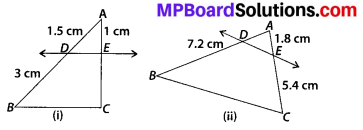Solution:
(i) Since DE || BC [Given]
∴ Using the Basic proportionality theorem,
We have $$\frac{A D}{D B}=\frac{A E}{E C}$$
Since, AD = 1.5 cm, DB = 3 cm and AE = 1 cm,
∴ $$\frac{1.5 \mathrm{cm}}{3 \mathrm{cm}}=\frac{1 \mathrm{cm}}{E C}$$
By cross-multiplication, we have
EC × 1.5 = 1 × 3
⇒ EC = $$\frac{1 \times 3}{1.5}=\frac{1 \times 3 \times 10}{15}$$
EC = 2 cm
(ii) In ∆ABC, DE || BC
Using the Basic proportionality theorem,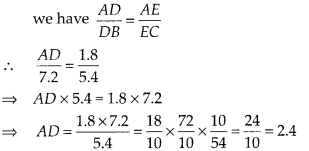Question 2.
E and F are points on the sides PQ and PR respectively of a ∆PQR. For each of the following cases, state whether EF || QR;
(i) PE = 3.9 cm, EQ = 3 cm, PF = 3.6 cm and FR = 2.4 cm
(ii) PE = 4 cm, QE = 4.5 cm, PF = 8 cm and RF = 9cm
(iii) PQ = 1.28 cm, PR = 2.56 cm, PE = 0.18 cm and PF = 0.36 cm
Solution:
(i) We have, PE = 3.9 cm, EQ = 3 cm, PF = 3.6 cm and FR = 2.4 cm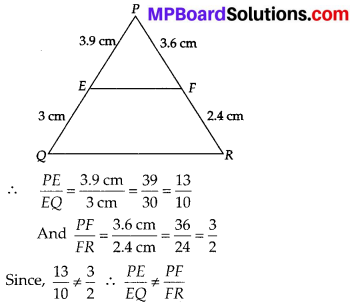⇒ EF is not parallel to QR.
(ii) We have, PE = 4 cm, QE = 4.5 cm PF = 8 cm and RF = 9 cm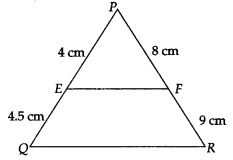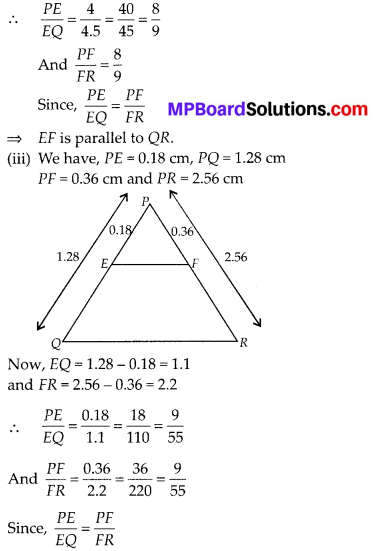⇒ EF is parallel to QR.Question 3.
In the figure, if LM || CB and LN || CD, prove that $$\frac{A M}{A B}=\frac{A N}{A D}$$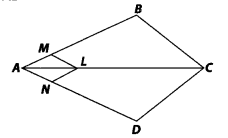Solution:
In ∆ABC, LM || CB [given]
∴ Using the Basic proportionality theorem, we have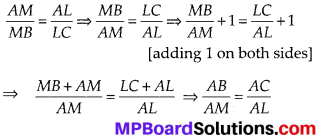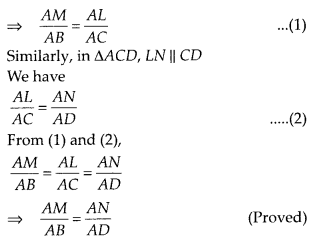Question 4.
In the figure, DE || ACand DF || AE.
Prove that $$\frac{B F}{F E}=\frac{B E}{E C}$$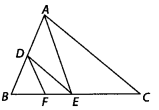Solution:
In ∆ABC
∵ DE || AC [given]
Using the basic proportionality theorem, we have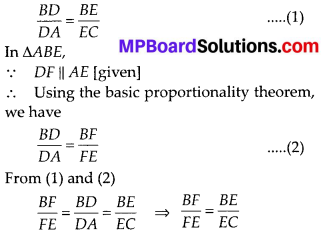Question 5.
In the figure, DE || OQ and DF || OR. Show that EF || QR.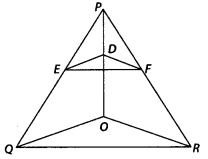Solution:
In ∆PQO,
∵ DE || OQ [given]
∴ Using the Basic proportionality theorem, we have
$$\frac{P E}{E Q}=\frac{P D}{D O}$$ …………… (1)
Again, in ∆POR, DF || OR [given]
∴ Using the Basic proportionality theorem, we have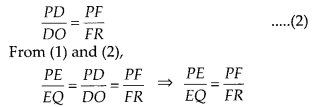Now, in ∆PQR,
∵ E and F are two distinct points on PQ and PR respectively and $$\frac{P E}{E Q}=\frac{P F}{F R}$$,
i.e., E and F divide the two sides PQ and PR of ∆PQR in the same ratio.
∴ By converse of Basic proportionality theorem, EF || QR.

Question 6.
In the figure A, B and C are points on OP, OQ and OR respectively such that AB || PQ and AC || PR. Show that BC || QR.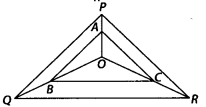Solution:
In ∆PQR, O is a point and OP, OQ and OR are joined. We have points A, B and C on OP, OQ and OR respectively such that AB || PQ and AC || PR.
Now, in ∆OPQ,
∵ AB || PQ [Given]
Using the Basic proportionality theorem, we have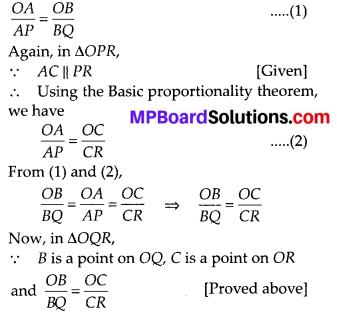i.e., B and C divide the sides OQ and OR of ∆OQR in the same ratio.
By converse of Basic proportionality theorem, BC || QR.Question 7.
Using Basic proportionality theorem, prove that a line drawn through the mid-point of one side of a triangle parallel to another side bisects the third side. A
Solution:
We have ∆ABC, in which D is the midpoint of AB and E is a point on AC such that DE || BC.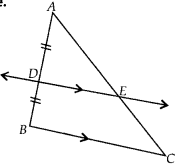∵ DE || BC [given]
∴ Using the Basic proportionality theorem, we get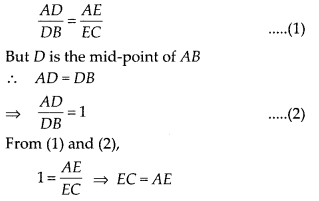⇒ E is the mid point of AC. Hence, it is proved that a line through the midpoint of one side of a triangle parallel to another side bisects the third side.

Question 8.
Using converse of basic proportionality theorem, prove that the line joining the mid-points of any two sides of a triangle is parallel to the third side.
Solution:
We have ∆ABC, in which D and E are the mid-points of sides AB and AC respectively.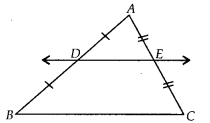∴ AD = DB ……………. (1)
and AE = EC ………….. (2)
From (1) and (2), we have
$$\frac{A D}{D B}$$ = 1 and $$\frac{A E}{E C}$$ = 1
⇒ $$\frac{A D}{D B}=\frac{A E}{E C}$$
⇒ DE || BC (By converse proportionality theorem).Question 9.
ABCD is a trapezium in which AB || DC and its diagonals intersect each other at the point O. Show that $$\frac{A O}{B O}=\frac{C O}{D O}$$.
Solution:
We have, a trapezium ABCD such that AB || DC. The diagonals AC and BD intersect each other at O.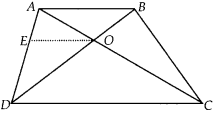Let us draw OE parallel to either AB or DC.
OE || DC [By construction]
∴ Using the Basic proportionality theorem, we get
$$\frac{A E}{E D}=\frac{A O}{C O}$$ …………. (1)
In ∆ABD
OE || AB [By construction]
∴ Using the Basic proportionality theorem, we get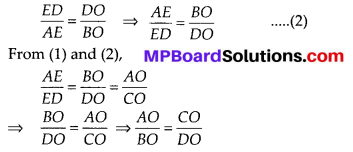Question 10.
The diagonals of a quadrilateral ABCD intersect each other at the point O such that $$\frac{A O}{B O}=\frac{C O}{D O}$$. Show that ABCD is a trapezium.
Solution:
It is given that $$\frac{A O}{B O}=\frac{C O}{D O}$$
From $$\frac{A O}{B O}=\frac{C O}{D O}$$, we have $$\frac{A O}{C O}=\frac{B O}{D O}$$
Let us draw OE such that OE || BA
In ∆ADB, OE || AB [By construction]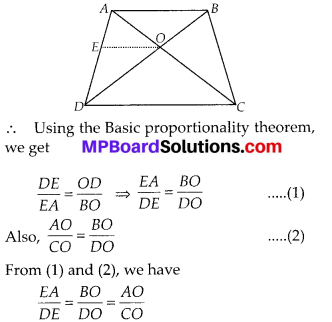i.e., the points O and E divide the sides AC and AD of ∆ADC respectively in the same ratio.
∴ Using the converse of Basic proportionality theorem, we get OE || DC and OE || AB
⇒ AB || DC
⇒ ABCD is a trapezium.